# Math Is Fun Forum

Discussion about math, puzzles, games and fun.   Useful symbols: ÷ × ½ √ ∞ ≠ ≤ ≥ ≈ ⇒ ± ∈ Δ θ ∴ ∑ ∫  π  -¹ ² ³ °

You are not logged in.

## #1 2006-10-04 17:56:17

Ricky
Moderator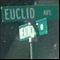Registered: 2005-12-04
Posts: 3,791

### Interesting proofs

Post any proof that you find interesting here.  It can be from any subject.  Below is one of my favorites:

"In the real world, this would be a problem.  But in mathematics, we can just define a place where this problem doesn't exist.  So we'll go ahead and do that now..."

Offline

## #2 2006-10-13 10:56:24

All_Is_Number
MemberRegistered: 2006-07-10
Posts: 258

### Re: Interesting proofs

I'm not saying that his proof was correct or incorrect, but....

I think he was saying that p=sqrt(2) and q=sqrt(2), so that p and q were both irrational, albeit equal.

You can shear a sheep many times but skin him only once.

Offline

## #3 2006-10-16 23:56:40

Dross
Member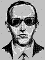Registered: 2006-08-24
Posts: 325

### Re: Interesting proofs

All_Is_Number wrote:

I'm not saying that his proof was correct or incorrect, but....

I think he was saying that p=sqrt(2) and q=sqrt(2), so that p and q were both irrational, albeit equal.

Yes, I realised that after I put that second post up. I was just having a bit of a no-brainer moment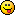Interesting proofs...

I always found Cantor's diagonal argument quite interesting, and you don't need a PhD to understand it.

Theorem:

It is impossible to write an exhaustive list of all real numbers.

Proof:

This is equivalent to saying that it is impossible to write an exhaustive list of all real numbers in (0,1). Let us suppose, on the contrary, that we can write such a list. It would be like:

Where each a[sub]ij[/sub] is one of the integers {0,1,...,9} representing the j[sup]th[/sup] decimal place of the i[sup]th[/sup] number in the list.

Now, this list is supposed to include all the real numbers between 0 and 1. But suppose we construct a number x with the following algorithm:

1) let the number be:

2) go down our previous list and look at the n[sup]th[/sup] digit of the n[sup]th[/sup] number.

3) if the n[sup]th[/sup] digit of the n[sup]th[/sup] number is 0, let the n[sup]th[/sup] digit of x be 1.

4) if the n[sup]th[/sup] digit of the n[sup]th[/sup] number is any number other than 0, let the n[sup]th[/sup] digit of x be 0.

We then have our number x well defined.

It is, however, not equal to the first number in our list, by the way we have constructed it (because at least one of the digits is different). It is also not equal to the second, or the third... or in fact any other number on our list.

Even if we put x at the start of our list, we can still construct another number using the same algorithm that would not be on the list, so our list will never be exhaustive.

Offline

## #4 2006-10-17 21:09:58

Zhylliolom
Real Member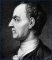Registered: 2005-09-05
Posts: 412

### Re: Interesting proofs

Theorem:

There are infinitely many primes.

Proof:

Define a topology on the set of integers by using the arithmetic progressions (from -∞ to ∞) as a basis.  It is easy to verify that this yields a topological space.  For each prime p let A[sub]p[/sub] consist of all multiples of p.  A[sub]p[/sub] is closed since its complement is the union of all the other arithmetic progressions with difference p.  Now let A be the union of the progressions A[sub]p[/sub].  If the number of primes is finite, then A is a finite union of closed sets, hence closed.  But all integers except -1 and 1 are multiples of some prime, so the complement of A is {-1, 1} which is obviously not open.  This shows A is not a finite union and there are infinitely many primes.

Last edited by Zhylliolom (2006-10-17 21:11:23)

Offline

## #5 2007-01-25 07:03:11

Ricky
ModeratorRegistered: 2005-12-04
Posts: 3,791

### Re: Interesting proofs

This proof is by Zhylliolom:

Prove that either:

or

For all natural numbers m and n.

Proof:  It is obvious that for any given natural numbers m and n, either

or

is true, since √2 is irrational and therefore cannot be equal to a rational number, and thus the result follows from trichotomy.

Now consider the inequality

where the ? denotes the unknown direction of the inequality. Since m and n are natural numbers, their sum is nonzero and positive, and thus we may multiply each side my m + n without reversing the inequality:

Once again, n is a natural number and thus is nonzero and positive, so we may divide each side by n and maintain the direction of the inequality:

Now subtract m/n and √2 from each side to obtain

which gives (after dividing through by 1 - √2, flipping the inequality, rationalizing, then flipping again so that we now have the original direction (these steps are left to the reader as an exercise))

which gives the direction of the inequality as the opposite of the "initial condition" m/n ¿ √2, where ¿ denotes the original (and opposite) inequality direction, so that if m/n < √2 then (m + 2n)/(m + n) > √2 or if m/n > √2 then (m + 2n)/(m + n) < √2.

Thus, either

or

is true for natural numbers m and n.

"In the real world, this would be a problem.  But in mathematics, we can just define a place where this problem doesn't exist.  So we'll go ahead and do that now..."

Offline

## #6 2007-01-25 07:13:06

Ricky
ModeratorRegistered: 2005-12-04
Posts: 3,791

### Re: Interesting proofs

Prove that card(A) < card(P(A)), where card(A) is the cardinality of A, and P(A) is the power set of A.

Proof:

Define a function f:A->P(A) such that f(a) = {a} for all a in A.  Thus, if a = b, then {a} = {b}, and so f(a) = f(b), which means that f is well defined.  Assume that f(a) = f(b).  Then {a} = {b}, and so for all x in {a}, x is in b.  Since a is in {a}, a is in {b}, and thus, a = b, as b is the only element in {b}.  So f is 1-1.  This proves that card(A) ≤ card(P(A)).

Now we wish to show that card(A) ≠ card(P(A)).  The equivalent of this is that there does not exist a bijective function from A to P(A).  Assume f is such a bijective function, so f is 1-1 and onto.  Define:

Note that it must be that:

And so T is in the range of f.  Thus, there exists some t such that f(t) = T.

First, assume that t is in T.  Then it must be that:

And so t is not in f(t) = T, so t is not in T.  Contradiciton.

Now assume that t is not in T.  Then it must be that:

So it must be that t is in f(t) = T, and so t is in T.  Contradiction.

Therefore, there must not exist such a function, and so card(A) < card(P(A)).

"In the real world, this would be a problem.  But in mathematics, we can just define a place where this problem doesn't exist.  So we'll go ahead and do that now..."

Offline

## #7 2007-02-03 21:48:33

Jai GaneshRegistered: 2005-06-28
Posts: 44,437

### Re: Interesting proofs

I had read somewhere that 26 is the only number in the universe jammed between a square and a cube.
Has anyone seen a proof of this, or can someone try to prove this?

It appears to me that if one wants to make progress in mathematics, one should study the masters and not the pupils. - Niels Henrik Abel.

Nothing is better than reading and gaining more and more knowledge - Stephen William Hawking.

Offline

## #8 2007-02-03 22:10:34

Toast
Real MemberRegistered: 2006-10-08
Posts: 1,321

### Re: Interesting proofs

I'm willing to bet that with the increase in squares and cubes as you move up the number line, there will be more numbers that share 26's properties, after all, we have the whole number line to exploreOffline

## #9 2007-02-03 22:31:14

Jai GaneshRegistered: 2005-06-28
Posts: 44,437

### Re: Interesting proofs

That couldn't have been said without a proof! I am sure there exists one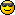It appears to me that if one wants to make progress in mathematics, one should study the masters and not the pupils. - Niels Henrik Abel.

Nothing is better than reading and gaining more and more knowledge - Stephen William Hawking.

Offline

## #10 2007-02-04 00:01:20

mathsyperson
Moderator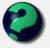Registered: 2005-06-22
Posts: 4,900

### Re: Interesting proofs

65536³ - 1 = 16777216² + 1Why did the vector cross the road?
It wanted to be normal.

Offline

## #11 2007-02-04 05:23:35

Ricky
ModeratorRegistered: 2005-12-04
Posts: 3,791

### Re: Interesting proofs

mathsyperson, I'm not sure what you're trying to do.  You want to find two numbers x and x+2 such that one is a square and one is a cube.  And yes, 26 is the only such number.

Ganesh, you probably saw that one these forums, there was a post not long ago.  The proof involves algebraic number theory.  I have a fair understanding of it, but it will be difficult to try to "dumb it down" (just an expression) as it were.  Give me a few days and I'll see what I can do.

Also, all these discussion posts will be deleted once the proof is up.

"In the real world, this would be a problem.  But in mathematics, we can just define a place where this problem doesn't exist.  So we'll go ahead and do that now..."

Offline

## #12 2007-02-04 05:54:00

mathsyperson
ModeratorRegistered: 2005-06-22
Posts: 4,900

### Re: Interesting proofs

Oh, sorry. I tried to brute-force it in Excel and it gave me that 65536³ and 16777216² were two apart. Something must have gone wrong with rounding errors. Apologies.Why did the vector cross the road?
It wanted to be normal.

Offline

## #13 2007-07-31 07:10:43

krassi_holmz
Real Member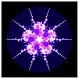Registered: 2005-12-02
Posts: 1,905

### Re: Interesting proofs

This is something like the Mihailescu's theorem: http://en.wikipedia.org/wiki/Mih%C4%83i … 7s_theorem

IPBLE:  Increasing Performance By Lowering Expectations.

Offline

## #14 2007-08-01 07:50:46

ben
Member
Registered: 2006-07-12
Posts: 106

### Re: Interesting proofs

Ricky wrote:

Prove that card(A) < card(P(A)), where card(A) is the cardinality of A, and P(A) is the power set of A.

Yes, your proof is good, I think, but Cantor's own proof was a lot sweeter, if you'll forgive me for saying so. Plus it requires (almost) no knowledge of mathematics!

You do know it, don't you? If not, and if anyone wants, I'll try and dredge it up from the memory bank.

Offline

## #15 2007-08-14 12:55:28

mikau
Member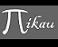Registered: 2005-08-22
Posts: 1,504

### Re: Interesting proofs

on the subject of interesting proofs, i was wondering..

could the output of a computer program be used as a proof for a theorem assuming each statement in the program were justified through sound logic or other theorems?

say for instance f(n) is an integer and we want to prove that the largest value of f(n), where n is some int between a and b, is equal to M.

say we write a simple for loop

``````int max = f(a);
for (int i = a +1; a <= b; i++)
{
if (f(i) > max )
max = f(i);
}
print(max);``````

if the program outputs M, could that be considered a valid proof that M is the max? Obviously we depend on the computer to make correct comparisons and to calculate f(n) exactly, but if we are willing to assume it does, could that be considered proof?

Last edited by mikau (2007-08-14 13:01:51)

A logarithm is just a misspelled algorithm.

Offline

## #16 2007-08-14 13:05:17

Ricky
ModeratorRegistered: 2005-12-04
Posts: 3,791

### Re: Interesting proofs

Yes, any problem with a finite number of computational cases can be proved using a computer.  For example, The Four Color Theorem.

"In the real world, this would be a problem.  But in mathematics, we can just define a place where this problem doesn't exist.  So we'll go ahead and do that now..."

Offline

## #17 2007-08-14 13:09:00

mikau
MemberRegistered: 2005-08-22
Posts: 1,504

### Re: Interesting proofs

that's COOL! still..they claim the proof is not satisfactory becuase it requires a computer. Hmm... just the oppinion of the writer, perhaps?

A logarithm is just a misspelled algorithm.

Offline

## #18 2007-08-14 13:50:51

Ricky
ModeratorRegistered: 2005-12-04
Posts: 3,791

### Re: Interesting proofs

The proof is most likely entirely valid, but it relies upon something we don't want to check, as it would be way too tedious.   That points to not something wrong with the proof, but with us instead (i.e. not being a computer, which would be way cool).  But many times in math, there are better proofs.  For example:

Prove that the square root of 2 is irrational.

Proof #1: Let √2 = m/n with gcd(m, n) = 1.  So 2 = m² / n², so 2n² = m², so m² is even and thus, m is even.  Since m is even, m² must be divisible by 4, and so n² even, so n is even.  Thus, gcd(n, m) > 1.  Contradiction.

Proof #2: Same as before, 2n² = m².  The number of prime factors of 2 in n² must be even (it's squared, all number of prime factors must be even).  Thus, on the left side there are an odd number of prime factors of 2.  With the same reasoning, there are an even number of prime factors in m².  Thus, it must be that 2n² ≠ m².  Contradiction.

Which proof is better?

And so the same goes with the proof in the link.  It's always better to have a proof that is straightforward rather than convoluted.

"In the real world, this would be a problem.  But in mathematics, we can just define a place where this problem doesn't exist.  So we'll go ahead and do that now..."

Offline

## #19 2007-11-25 07:59:49

mikau
MemberRegistered: 2005-08-22
Posts: 1,504

### Re: Interesting proofs

I'm really happy I managed to figure out this proof last night, turned out it was exactly what my book did and I thought it was kind of cool.

suppose

are linearly dependent.
now suppose we one by one remove every vector in

that can be expressed as a linear combination of the others. When we are done we will have a linearly independent subset of

consisting of m elements, call it

we have the set

is not empty for the vectors are all non zero so the linearly independent subset contains at least one element.

Now take an element

not in the set

we have that

but also we have that
can be expressed as a linear combination of

so

where

are the scalers of the linear combination. But also by the distributive property of matrix multiplication:

thus

so

since

are linearly independent, we have that all scalers are zero.
Recall now that we have all eigenvalues are unique, and

is not an element of

so

thus

thus, since they are linearly dependent, and by the zero factor theorem, all scalers

must be zero. But this implies that

and we were given all vectors were nonzero. Hence the contradiction.
So it must be linearly independent.

Last edited by mikau (2007-11-25 13:33:12)

A logarithm is just a misspelled algorithm.

Offline

## #20 2007-11-25 08:12:00

luca-deltodesco
MemberRegistered: 2006-05-05
Posts: 1,470

### Re: Interesting proofs

to do with computer proof, people dont want to trust that the computer program was correct because it wasn't done by a human, but to be honest i would more likely trust a well written computer program than a team of humans running through thousands upon thousands of scenarios where they would likely become lazy and make mistakesThe Beginning Of All Things To End.
The End Of All Things To Come.

Offline

## #21 2007-11-25 10:37:10

Ricky
ModeratorRegistered: 2005-12-04
Posts: 3,791

### Re: Interesting proofs

But the question is, do you realize what it is you are trusting when you trust a computer?

I write a program in some high level language, say it's Java or C++.  First off, you have to trust my code.  This is very difficult to check for correctness when it comes to complex programs.  But in the pipeline that is execution, it is the easiest part to check.  Next thing you have to trust is the compiler.  You need to trust it to read my code properly, run it's algorithm, and spit out the proper machine code.  Compiler programming is one of the most difficult types of programming there is, and almost everyone says that it's closest rival: OS programming, it really no match.  There could be bugs anywhere in the compiler itself or in any one of the libraries which are used to compile the code.  The next thing you have to trust is the OS, which typically handles many operations when it comes to a program.  At the very least, it is responsible for loading the program, but one must also not forget things such as process switches which happen on any given machine today.  The only thing left after this is the hardware itself, which we know errors do occur.  Whether they occur because of a design flaw, wear and tear, or a neutrino gets the 1 in a million billion trillion chance of hitting my ram chip, we are certain that they do in fact occur.

I'm not saying that we shouldn't trust computers.  All I'm saying is that one should be very knowledgeable of what he puts his trust in.

"In the real world, this would be a problem.  But in mathematics, we can just define a place where this problem doesn't exist.  So we'll go ahead and do that now..."

Offline

## #22 2007-11-25 10:56:05

mathsyperson
ModeratorRegistered: 2005-06-22
Posts: 4,900

### Re: Interesting proofs

The human brain can be viewed as a very complex computer, and we have no idea how it works.
Does that mean that everything we know is wrong?Why did the vector cross the road?
It wanted to be normal.

Offline

## #23 2007-11-25 13:30:58

mikau
MemberRegistered: 2005-08-22
Posts: 1,504

### Re: Interesting proofs

blah, just fixed the latex in my proof. Have a look!A logarithm is just a misspelled algorithm.

Offline

## #24 2007-12-06 07:55:58

100'
Member
Registered: 2007-12-06
Posts: 8

### Re: Interesting proofs

First off, hiya.

Second, 26 is in fact the only number which can be written as a square plus one, cube minus one. This was proven by Fermat.

Thirdly, regarding the outputs of computers being considered proof, the four colour theorem (mentioned earlier) is a good example. The solution is frowned upon as aesthetics is always desired in proof. Unfortunately the approach used on the four colour theorem required testing thousands of possible combinations once the original map had been reduced. An interesting note is that Turing proved (indirectly) that a machine (human or mechanical) will never be able to decide whether any given theorem is provable or not (this was one of Hilberts problems proposed in 1900 and ties in almost directly with Godels incompleteness theorem).

Finally, the proof that I find most interesting is Liebniz's infinite sequence converging to pi/4 (I have no real notation software and I am new to the forums so I am not sure what everything means =p so let (b,a)∫f(x) dx be the integral of f(x) with respect to x between a and b.

Basic calculus shows:
arctan(b) = (b,0)∫[1/(1+x^2)]dx
It follows that
arctan(1) = (1,0)∫[1/(1+x^2)]dx = pi/4

Now look at the formula for the sum to infinity of a geometric progression:
1+q+q^2+q^3.... = 1/(1-q)

By inspection it is clear that:
1/(1+x^2) = 1-x^2+x^4-x^6...

Integrating this new expression between 1 and 0 term by term gives:
pi/4 = 1 - 1/3 + 1/5 - 1/7 + 1/9....

I just thought it was rather elegant.Offline

## #25 2011-01-08 08:23:25

4DLiVing
Member
Registered: 2011-01-08
Posts: 22

### Re: Interesting proofs

ganesh,

Your post on whether or not 26 was the only number jammed between a square and a cube intrigued me.

My investigation has only brought me more questions.... here goes.

Well, I approached this problem like this:

can I find an x and a y, such that:

(a) x^2 + 1 = y^3 - 1    OR     (b) x^2 - 1 = y^3 + 1.

I solved (a) for y to get y = (x^2 + 2)^(1/3) and similaraly (b) became y = (x^2 - 2)^(1/3).

I set the domain for natural numbers in excel and plugged these equations in.

(a), from x = 1 to x = 10,000 returns only 1 rational solution (5, 3) and indeed
5^2 + 1 = 26 = 3^3 - 1
(b), from x = 1 to x = 10,000 returned only 1 rational solution (1, -1) and indeed
1^2 - 1 = 0 = (-1)^3 + 1.

So if we look at both sides of the coin, there appears to be two numbers "jammed" between a square and a cube: 26 and 0.

Now this does suggest that if you want the square and cube to be natural numbers, then 26 is the only solution.

But if you say the square and the cube can be integers, then 26 and 0 are solutions.

Furthurmore, if we say the square and cube can be irrational numbers, then we have infinite solutions as the functions show us.  An example would be the solution (sqrt(6), 2) for (a) where (sqrt(6))^2 + 1 = 7 = 2^3 - 1.

Another interesting tidbit: as x got very high, the difference between the (a) and (b) functions seemed to be approaching zero.  Maybe the + 1 and - 1 become negligible as the values of x^2 and y^3 get into the tens of millions and they will not impact the value of x^2 and y^3 enough to cause for the situation we are looking for.

This is far from a proof, but my investigation i feel has offered some great insights as to how we may formally prove this situation.  It seems though that this is the theorem we would like to prove:

Prove that, for all natural numbers, 26 is the only number between a square and a cube, or

Prove that, for all integers, 26 and 0 are the only numbers between a square and a cube.

Let me know what you think!

Offline